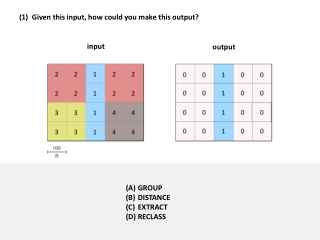# (1) Given this input, how could you make this output? - PowerPoint PPT PresentationDownload Presentation(1) Given this input, how could you make this output?

(1) Given this input, how could you make this output?Download Presentation## (1) Given this input, how could you make this output?

- - - - - - - - - - - - - - - - - - - - - - - - - - - E N D - - - - - - - - - - - - - - - - - - - - - - - - - - -
##### Presentation Transcript

1. (1) Given this input, how could you make this output? input output GROUP DISTANCE EXTRACT RECLASS

2. (2) Given this input, how could you make this output? input output GROUP DISTANCE EXTRACT RECLASS

3. (3) Given this input, how could you make this output? input output GROUP DISTANCE EXTRACT RECLASS

4. (4) Given these inputs: What would the output look like?

5. (5) If you used this input to make this output: How would you have defined the RECLASS table:

6. (6) If you made the output on the right with DISTANCE: output Which layer or layers could you have used as input? 1 only 2 only 1 or 3 1, 2 or 3

7. (7) Given these inputs: What would the output look like?

8. (8) Given this input, how would you make this output? input output • RECLASS • GROUP • First use GROUP, then RECLASS that output • First use RECLASS, then GROUP that output

9. (9) Given this input, how could you make this output? input output DISTANCE RECLASS First GROUP, then DISTANCE First RECLASS, then DISTANCE

10. (10) Which problem(s) could you solve with the steps that you learned in the tutorial? Problem 1. Urban planners suggest that not more than 9% of the land in any given area should be allocated for parking. Given a layer of parking lots and a layer of parking zones on a college campus, please use a GIS to calculate how much land in each parking zone is used as a parking lot. Problem 2. Island biogeography theory expects islands that are far from the mainland to have fewer species than islands that are near the mainland. Given a layer that represents the mainland and another layer that represents islands, please use a GIS to calculate the distance of each island from the mainland. Problem 1 only Problem 2 only Problems 1 and 2 Neither